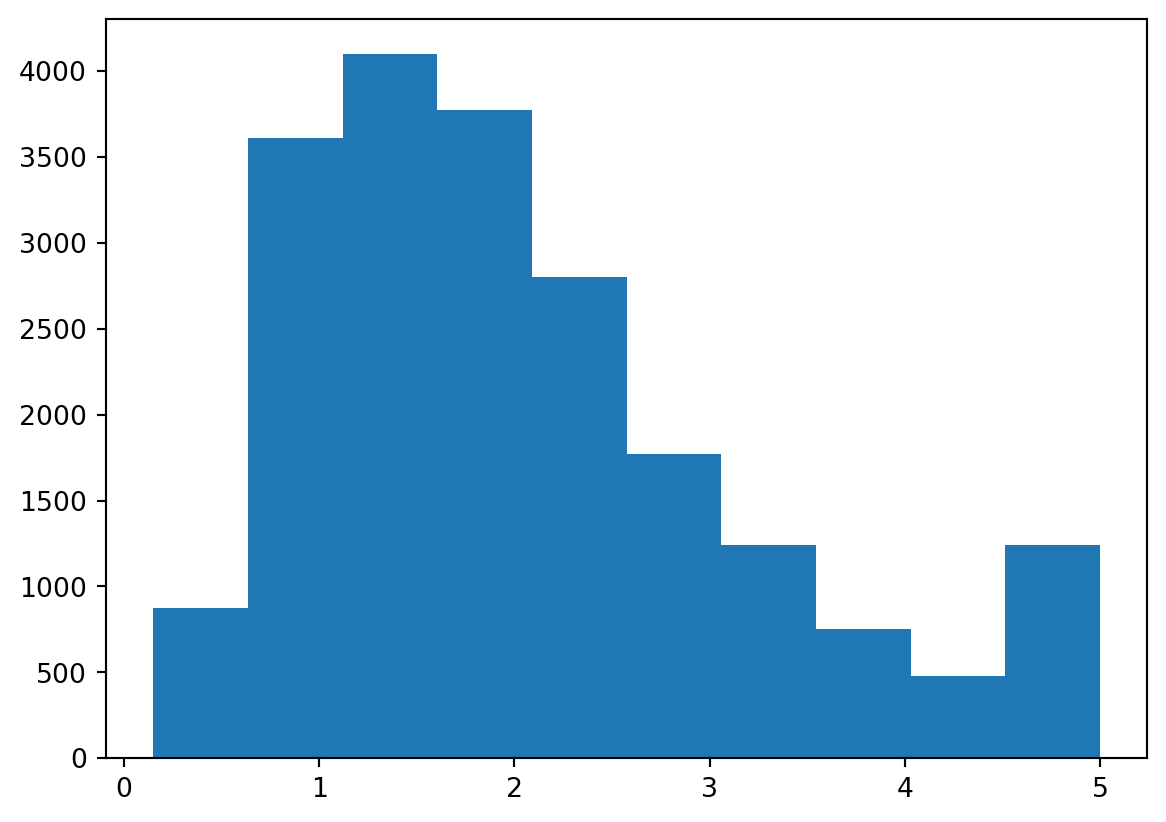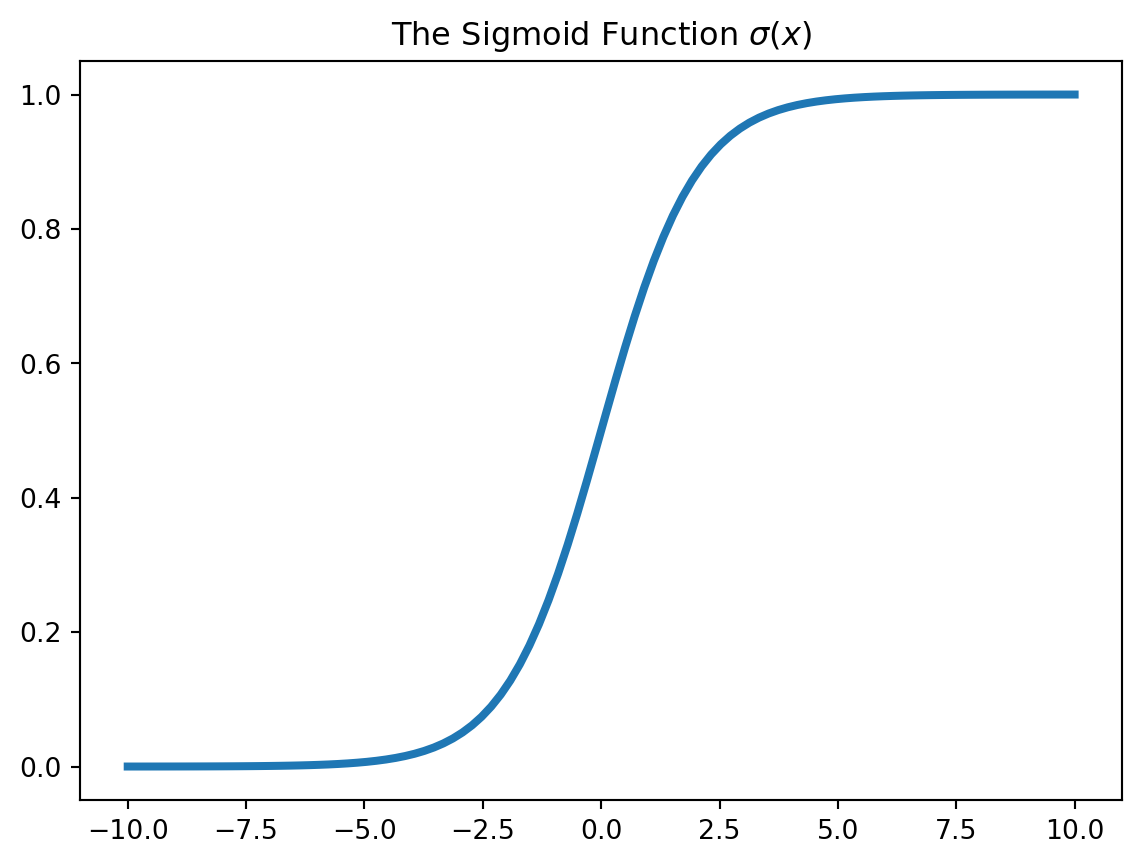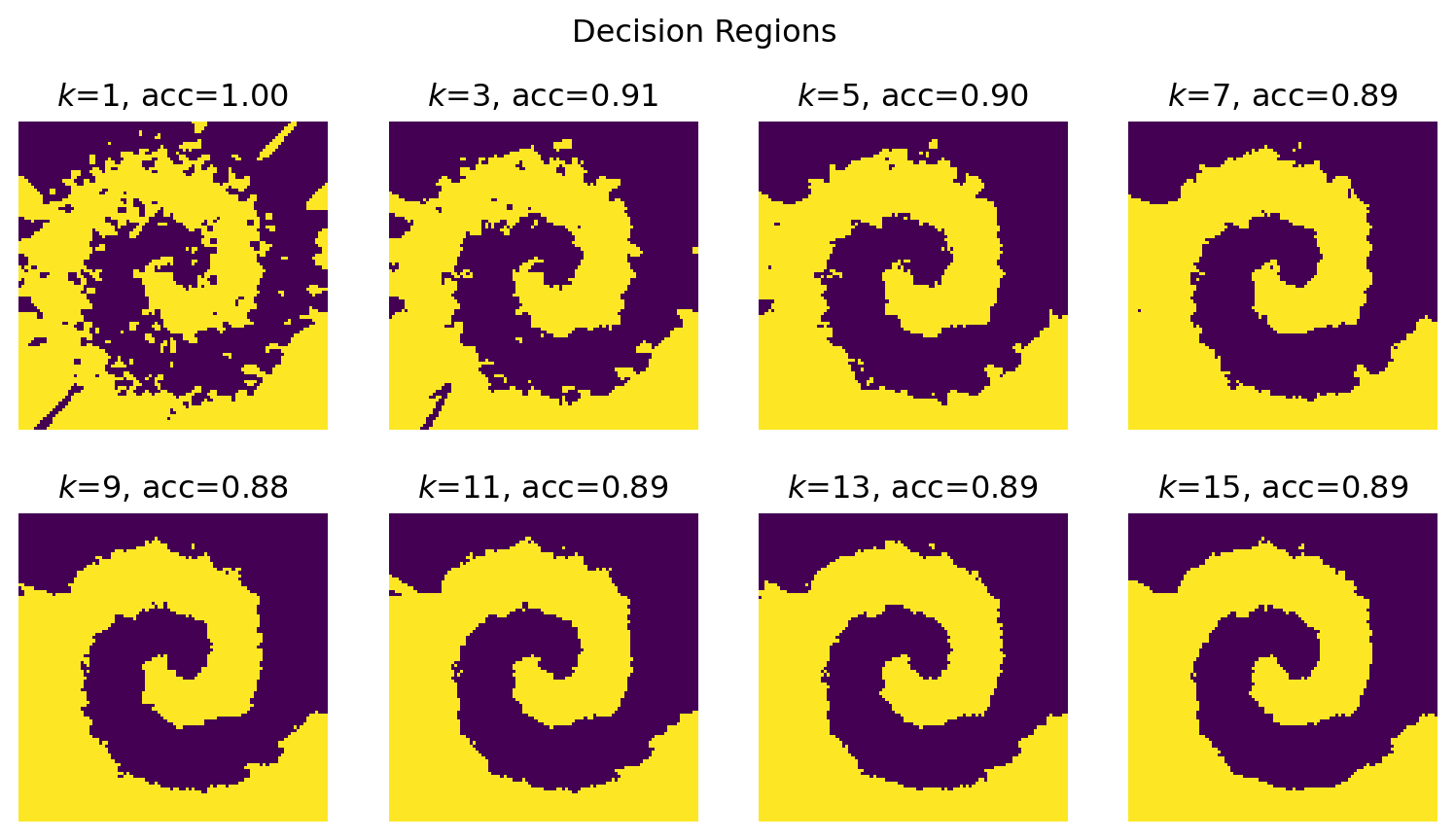# Sklearn workflow

Author

Marie-Hélène Burle

Scikit-learn has a very clean and consistent API, making it very easy to use: a similar workflow can be applied to most techniques. Let’s go over two examples.

This code was modified from Matthew Greenberg.

from sklearn.datasets import fetch_california_housing, load_breast_cancer
from sklearn.linear_model import LinearRegression, LogisticRegression
from sklearn.ensemble import RandomForestRegressor
from sklearn.neighbors import KNeighborsClassifier
from sklearn.metrics import mean_squared_error, mean_absolute_percentage_error, accuracy_score

import pandas as pd

import matplotlib
from matplotlib import pyplot as plt

import numpy as np

from collections import Counter

## Example 1: California housing dataset

### Load and explore the data

cal_housing = fetch_california_housing()
type(cal_housing)
sklearn.utils._bunch.Bunch

Let’s look at the attributes of cal_housing:

dir(cal_housing)
['DESCR', 'data', 'feature_names', 'frame', 'target', 'target_names']
cal_housing.feature_names
['MedInc',
'HouseAge',
'AveRooms',
'AveBedrms',
'Population',
'AveOccup',
'Latitude',
'Longitude']
print(cal_housing.DESCR)
.. _california_housing_dataset:

California Housing dataset
--------------------------

**Data Set Characteristics:**

:Number of Instances: 20640

:Number of Attributes: 8 numeric, predictive attributes and the target

:Attribute Information:
- MedInc        median income in block group
- HouseAge      median house age in block group
- AveRooms      average number of rooms per household
- AveBedrms     average number of bedrooms per household
- Population    block group population
- AveOccup      average number of household members
- Latitude      block group latitude
- Longitude     block group longitude

:Missing Attribute Values: None

This dataset was obtained from the StatLib repository.
https://www.dcc.fc.up.pt/~ltorgo/Regression/cal_housing.html

The target variable is the median house value for California districts,
expressed in hundreds of thousands of dollars (100,000). This dataset was derived from the 1990 U.S. census, using one row per census block group. A block group is the smallest geographical unit for which the U.S. Census Bureau publishes sample data (a block group typically has a population of 600 to 3,000 people). A household is a group of people residing within a home. Since the average number of rooms and bedrooms in this dataset are provided per household, these columns may take surprisingly large values for block groups with few households and many empty houses, such as vacation resorts. It can be downloaded/loaded using the :func:sklearn.datasets.fetch_california_housing function. .. topic:: References - Pace, R. Kelley and Ronald Barry, Sparse Spatial Autoregressions, Statistics and Probability Letters, 33 (1997) 291-297  X = cal_housing.data y = cal_housing.target This can also be obtained with X, y = fetch_california_housing(return_X_y=True). Let’s have a look at the shape of X and y: X.shape (20640, 8) y.shape (20640,) While not at all necessary, we can turn this bunch object into a more familiar data frame to explore the data further: cal_housing_df = pd.DataFrame(cal_housing.data, columns=cal_housing.feature_names) cal_housing_df.head() MedInc HouseAge AveRooms AveBedrms Population AveOccup Latitude Longitude 0 8.3252 41.0 6.984127 1.023810 322.0 2.555556 37.88 -122.23 1 8.3014 21.0 6.238137 0.971880 2401.0 2.109842 37.86 -122.22 2 7.2574 52.0 8.288136 1.073446 496.0 2.802260 37.85 -122.24 3 5.6431 52.0 5.817352 1.073059 558.0 2.547945 37.85 -122.25 4 3.8462 52.0 6.281853 1.081081 565.0 2.181467 37.85 -122.25 cal_housing_df.tail() MedInc HouseAge AveRooms AveBedrms Population AveOccup Latitude Longitude 20635 1.5603 25.0 5.045455 1.133333 845.0 2.560606 39.48 -121.09 20636 2.5568 18.0 6.114035 1.315789 356.0 3.122807 39.49 -121.21 20637 1.7000 17.0 5.205543 1.120092 1007.0 2.325635 39.43 -121.22 20638 1.8672 18.0 5.329513 1.171920 741.0 2.123209 39.43 -121.32 20639 2.3886 16.0 5.254717 1.162264 1387.0 2.616981 39.37 -121.24 cal_housing_df.info() <class 'pandas.core.frame.DataFrame'> RangeIndex: 20640 entries, 0 to 20639 Data columns (total 8 columns): # Column Non-Null Count Dtype --- ------ -------------- ----- 0 MedInc 20640 non-null float64 1 HouseAge 20640 non-null float64 2 AveRooms 20640 non-null float64 3 AveBedrms 20640 non-null float64 4 Population 20640 non-null float64 5 AveOccup 20640 non-null float64 6 Latitude 20640 non-null float64 7 Longitude 20640 non-null float64 dtypes: float64(8) memory usage: 1.3 MB cal_housing_df.describe()  MedInc HouseAge AveRooms AveBedrms Population AveOccup Latitude Longitude count 20640.000000 20640.000000 20640.000000 20640.000000 20640.000000 20640.000000 20640.000000 20640.000000 mean 3.870671 28.639486 5.429000 1.096675 1425.476744 3.070655 35.631861 -119.569704 std 1.899822 12.585558 2.474173 0.473911 1132.462122 10.386050 2.135952 2.003532 min 0.499900 1.000000 0.846154 0.333333 3.000000 0.692308 32.540000 -124.350000 25% 2.563400 18.000000 4.440716 1.006079 787.000000 2.429741 33.930000 -121.800000 50% 3.534800 29.000000 5.229129 1.048780 1166.000000 2.818116 34.260000 -118.490000 75% 4.743250 37.000000 6.052381 1.099526 1725.000000 3.282261 37.710000 -118.010000 max 15.000100 52.000000 141.909091 34.066667 35682.000000 1243.333333 41.950000 -114.310000 We can even plot it: plt.hist(y) (array([ 877., 3612., 4099., 3771., 2799., 1769., 1239., 752., 479., 1243.]), array([0.14999 , 0.634992, 1.119994, 1.604996, 2.089998, 2.575 , 3.060002, 3.545004, 4.030006, 4.515008, 5.00001 ]), <BarContainer object of 10 artists>)### Create and fit a model Let’s start with a very simple model: linear regression. model = LinearRegression().fit(X, y) This is equivalent to: model = LinearRegression() model.fit(X, y) First, we create an instance of the class LinearRegression, then we call .fit() on it to fit the model. model.coef_ array([ 4.36693293e-01, 9.43577803e-03, -1.07322041e-01, 6.45065694e-01, -3.97638942e-06, -3.78654265e-03, -4.21314378e-01, -4.34513755e-01]) Trailing underscores indicate that an attribute is estimated. .coef_ here is an estimated value. model.coef_.shape (8,) model.intercept_ -36.94192020718425 We can now get our predictions: y_hat = model.predict(X) And calculate some measures of error: • Sum of squared errors np.sum((y - y_hat) ** 2) 10821.985154850292 • Mean squared error mean_squared_error(y, y_hat) 0.5243209861846072 MSE could also be calculated with np.mean((y - y_hat)**2). mean_absolute_percentage_error(y, y_hat) 0.31715404597233504 Index of minimum value: model.coef_.argmin() 7 Index of maximum value: model.coef_.argmax() 3 XX = np.concatenate([np.ones((len(X), 1)), X], axis=1) beta = np.linalg.lstsq(XX, y, rcond=None) intercept_, *coef_ = beta intercept_, model.intercept_ (-36.94192020718431, -36.94192020718425) np.allclose(coef_, model.coef_) True This means that the two arrays are equal element-wise, within a certain tolerance. X_test = np.random.normal(size=(10, X.shape)) X_test.shape (10, 8) y_test = X_test @ coef_ + intercept_ y_test array([-35.66108585, -36.99366322, -38.38641628, -37.80068633, -37.75440718, -36.94613883, -36.23397014, -37.09329031, -37.74423072, -36.33948053]) model.predict(X_test) array([-35.66108585, -36.99366322, -38.38641628, -37.80068633, -37.75440718, -36.94613883, -36.23397014, -37.09329031, -37.74423072, -36.33948053]) Of course, instead of LinearRegression(), we could have used another model such as a random forest regressor (a meta estimator that fits a number of classifying decision trees on various sub-samples of the dataset and uses averaging to improve the predictive accuracy and control over-fitting) for instance: model = RandomForestRegressor().fit(X, y).predict(X_test) model array([1.53359 , 1.43435 , 1.5799001, 1.5324 , 1.5799001, 1.5116701, 1.5799001, 1.51034 , 1.5255501, 1.5799001]) Which is equivalent to: model = RandomForestRegressor() model.fit(X, y).predict(X_test) ## Example 2: breast cancer ### Load and explore the data b_cancer = load_breast_cancer() Let’s print the description of this dataset: print(b_cancer.DESCR) .. _breast_cancer_dataset: Breast cancer wisconsin (diagnostic) dataset -------------------------------------------- **Data Set Characteristics:** :Number of Instances: 569 :Number of Attributes: 30 numeric, predictive attributes and the class :Attribute Information: - radius (mean of distances from center to points on the perimeter) - texture (standard deviation of gray-scale values) - perimeter - area - smoothness (local variation in radius lengths) - compactness (perimeter^2 / area - 1.0) - concavity (severity of concave portions of the contour) - concave points (number of concave portions of the contour) - symmetry - fractal dimension ("coastline approximation" - 1) The mean, standard error, and "worst" or largest (mean of the three worst/largest values) of these features were computed for each image, resulting in 30 features. For instance, field 0 is Mean Radius, field 10 is Radius SE, field 20 is Worst Radius. - class: - WDBC-Malignant - WDBC-Benign :Summary Statistics: ===================================== ====== ====== Min Max ===================================== ====== ====== radius (mean): 6.981 28.11 texture (mean): 9.71 39.28 perimeter (mean): 43.79 188.5 area (mean): 143.5 2501.0 smoothness (mean): 0.053 0.163 compactness (mean): 0.019 0.345 concavity (mean): 0.0 0.427 concave points (mean): 0.0 0.201 symmetry (mean): 0.106 0.304 fractal dimension (mean): 0.05 0.097 radius (standard error): 0.112 2.873 texture (standard error): 0.36 4.885 perimeter (standard error): 0.757 21.98 area (standard error): 6.802 542.2 smoothness (standard error): 0.002 0.031 compactness (standard error): 0.002 0.135 concavity (standard error): 0.0 0.396 concave points (standard error): 0.0 0.053 symmetry (standard error): 0.008 0.079 fractal dimension (standard error): 0.001 0.03 radius (worst): 7.93 36.04 texture (worst): 12.02 49.54 perimeter (worst): 50.41 251.2 area (worst): 185.2 4254.0 smoothness (worst): 0.071 0.223 compactness (worst): 0.027 1.058 concavity (worst): 0.0 1.252 concave points (worst): 0.0 0.291 symmetry (worst): 0.156 0.664 fractal dimension (worst): 0.055 0.208 ===================================== ====== ====== :Missing Attribute Values: None :Class Distribution: 212 - Malignant, 357 - Benign :Creator: Dr. William H. Wolberg, W. Nick Street, Olvi L. Mangasarian :Donor: Nick Street :Date: November, 1995 This is a copy of UCI ML Breast Cancer Wisconsin (Diagnostic) datasets. https://goo.gl/U2Uwz2 Features are computed from a digitized image of a fine needle aspirate (FNA) of a breast mass. They describe characteristics of the cell nuclei present in the image. Separating plane described above was obtained using Multisurface Method-Tree (MSM-T) [K. P. Bennett, "Decision Tree Construction Via Linear Programming." Proceedings of the 4th Midwest Artificial Intelligence and Cognitive Science Society, pp. 97-101, 1992], a classification method which uses linear programming to construct a decision tree. Relevant features were selected using an exhaustive search in the space of 1-4 features and 1-3 separating planes. The actual linear program used to obtain the separating plane in the 3-dimensional space is that described in: [K. P. Bennett and O. L. Mangasarian: "Robust Linear Programming Discrimination of Two Linearly Inseparable Sets", Optimization Methods and Software 1, 1992, 23-34]. This database is also available through the UW CS ftp server: ftp ftp.cs.wisc.edu cd math-prog/cpo-dataset/machine-learn/WDBC/ .. topic:: References - W.N. Street, W.H. Wolberg and O.L. Mangasarian. Nuclear feature extraction for breast tumor diagnosis. IS&T/SPIE 1993 International Symposium on Electronic Imaging: Science and Technology, volume 1905, pages 861-870, San Jose, CA, 1993. - O.L. Mangasarian, W.N. Street and W.H. Wolberg. Breast cancer diagnosis and prognosis via linear programming. Operations Research, 43(4), pages 570-577, July-August 1995. - W.H. Wolberg, W.N. Street, and O.L. Mangasarian. Machine learning techniques to diagnose breast cancer from fine-needle aspirates. Cancer Letters 77 (1994) 163-171. b_cancer.feature_names array(['mean radius', 'mean texture', 'mean perimeter', 'mean area', 'mean smoothness', 'mean compactness', 'mean concavity', 'mean concave points', 'mean symmetry', 'mean fractal dimension', 'radius error', 'texture error', 'perimeter error', 'area error', 'smoothness error', 'compactness error', 'concavity error', 'concave points error', 'symmetry error', 'fractal dimension error', 'worst radius', 'worst texture', 'worst perimeter', 'worst area', 'worst smoothness', 'worst compactness', 'worst concavity', 'worst concave points', 'worst symmetry', 'worst fractal dimension'], dtype='<U23') b_cancer.target_names array(['malignant', 'benign'], dtype='<U9') X = b_cancer.data y = b_cancer.target Here again, we could have used instead X, y = load_breast_cancer(return_X_y=True). X.shape (569, 30) y.shape (569,) set(y) {0, 1} Counter(y) Counter({1: 357, 0: 212}) ### Create and fit a first model model = LogisticRegression(max_iter=10000) y_hat = model.fit(X, y).predict(X) Get some measure of accuracy: accuracy_score(y, y_hat) 0.9578207381370826 This can also be obtained with: np.mean(y_hat == y) def sigmoid(x): return 1/(1 + np.exp(-x)) x = np.linspace(-10, 10, 100) plt.plot(x, sigmoid(x), lw=3) plt.title("The Sigmoid Function\\sigma(x)$") Text(0.5, 1.0, 'The Sigmoid Function$\\sigma(x)$')y_pred = 1*(sigmoid(X @ model.coef_.squeeze() + model.intercept_) > 0.5) assert np.all(y_pred == model.predict(X)) np.allclose( model.predict_proba(X)[:, 1], sigmoid(X @ model.coef_.squeeze() + model.intercept_) ) True def make_spirals(k=20, s=1.0, n=2000): X = np.zeros((n, 2)) y = np.round(np.random.uniform(size=n)).astype(int) r = np.random.uniform(size=n)*k*np.pi rr = r**0.5 theta = rr + np.random.normal(loc=0, scale=s, size=n) theta[y == 1] = theta[y == 1] + np.pi X[:,0] = rr*np.cos(theta) X[:,1] = rr*np.sin(theta) return X, y X, y = make_spirals() cmap = matplotlib.colormaps["viridis"] a = cmap(0) a = [*a[:3], 0.3] b = cmap(0.99) b = [*b[:3], 0.3] plt.figure(figsize=(7,7)) ax = plt.gca() ax.set_aspect("equal") ax.plot(X[y == 0, 0], X[y == 0, 1], 'o', color=a, ms=8, label="$y=0$") ax.plot(X[y == 1, 0], X[y == 1, 1], 'o', color=b, ms=8, label="$y=1$") plt.title("Spirals") plt.legend() <matplotlib.legend.Legend at 0x7f25dd9bd9d0>### Create and fit a second model Here, we use a logistic regression: model = LogisticRegression() y_hat = model.fit(X, y).predict(X) accuracy_score(y, y_hat) 0.5895 u = np.linspace(-8, 8, 100) v = np.linspace(-8, 8, 100) U, V = np.meshgrid(u, v) UV = np.array([U.ravel(), V.ravel()]).T U.shape, V.shape, UV.shape ((100, 100), (100, 100), (10000, 2)) np.ravel returns a contiguous flattened array. W = model.predict(UV).reshape(U.shape) W.shape (100, 100) plt.pcolormesh(U, V, W) <matplotlib.collections.QuadMesh at 0x7f25dd6c5b90>### Create and fit a third model Let’s use a k-nearest neighbours classifier this time: model = KNeighborsClassifier(n_neighbors=5) y_hat = model.fit(X, y).predict(X) accuracy_score(y, y_hat) 0.893 u = np.linspace(-8, 8, 100) v = np.linspace(-8, 8, 100) U, V = np.meshgrid(u, v) UV = np.array([U.ravel(), V.ravel()]).T U.shape, V.shape, UV.shape ((100, 100), (100, 100), (10000, 2)) W = model.predict(UV).reshape(U.shape) W.shape (100, 100) plt.pcolormesh(U, V, W) <matplotlib.collections.QuadMesh at 0x7f25dd8cb650>We can iterate over various values of k to see how the accuracy and pseudocolor plot evolve: fig, axes = plt.subplots(2, 4, figsize=(9.8, 5)) fig.suptitle("Decision Regions") u = np.linspace(-8, 8, 100) v = np.linspace(-8, 8, 100) U, V = np.meshgrid(u, v) UV = np.array([U.ravel(), V.ravel()]).T ks = np.arange(1, 16, 2) for k, ax in zip(ks, axes.ravel()): model = KNeighborsClassifier(n_neighbors=k) model.fit(X, y) acc = accuracy_score(y, model.predict(X)) W = model.predict(UV).reshape(U.shape) ax.imshow(W, origin="lower", cmap=cmap) ax.set_axis_off() ax.set_title(f"$k\$={k}, acc={acc:.2f}")